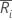Select the method or formula of your choice.

## Plotted points

Each plotted point, ri, represents the range for subgroup i.

## Center line

The value of the center line for each subgroup,, is calculated as follows:

### Notation

TermDescription
ni number of observations in subgroup i
d2(·)value of unbiasing constant d2 that corresponds to the value specified in parentheses.
σprocess standard deviation

## Control limits

### Lower control limit (LCL)

The value of the lower control limit for each subgroupis equal to the greater of the following:

or

### Upper control limit (UCL)

The value of the upper control limit for each subgroupis calculated as follows:

### Notation

TermDescription
d2(·)value of unbiasing constant d2 that corresponds to the value specified in parentheses.
ninumber of observations in subgroup i
σprocess standard deviation
kparameter for Test 1 (The default is 3.)
d3(·)value of unbiasing constant d3 that corresponds to the value specified in parentheses.